# Almost-prime number

Jump to: navigation, search

A natural numberof the formwhere the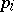are prime numbers and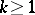is a constant. Prime numbers are the special case of almost-prime numbers for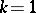. There are theorems for almost-prime numbers that generalize theorems on the distribution of prime numbers in the set of natural numbers. Several additive problems that have not yet been solved for prime numbers have been solved for almost-prime numbers.

#### Comments

See also Sieve method.

An example of a result on the distribution of almost-prime numbers generalizing the corresponding one on prime numbers is the following. Let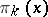be the number of square-free almost-prime numbers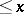. Then (cf. [a1], Sect. 22.18)